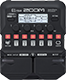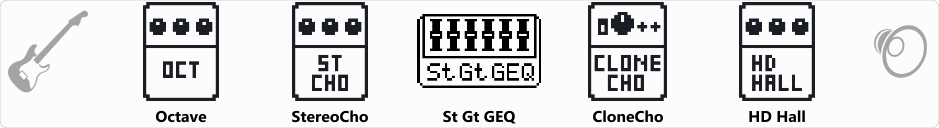# BASSTEREO

Discussion in 'Zoom G1/G1X Four' started by Ruling, Dec 4, 2020.

1. BASSTEREODevice: Zoom G1 Four
Firmware: 1.00

Name on device: BASSTEREO
Optimized for: Phones/Speaker

Effects chain:A kind of synth bass

Effect: "Octave" (Modulation), active - "yes"
"OCT1" = 100
"OCT2" = 15
"Tone" = 7
"Dry" = 100

Effect: "StereoCho" (Modulation), active - "yes"
"Depth" = 80
"Rate" = 30
"Tone" = 7
"Mix" = 60

Effect: "St Gt GEQ" (Filter), active - "yes"
"160" = 0.0
"400" = 0.0
"800" = 0.0
"3.2k" = 0.0
"6.4k" = 0.0
"12k" = 0.0
"VOL" = 80

Effect: "CloneCho" (Modulation), active - "yes"
"Depth" = 2
"Rate" = 23
"Tone" = 100
"Mix" = 100

Effect: "HD Hall" (Reverb), active - "yes"
"PreD" = 81
"Decay" = 45
"Mix" = 62
"Tail" = Off

Patch Volume: 50